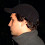### Basics on structured learning and prediction

I just pushed some of my structured learning code to github and hope that some people might find it useful. Before describing my code here, I wanted to give a basic intro into structured prediction. I hope I can at least convey some intuition for this vast research area. So here goes...

What is structured learning and prediction?

Structured prediction is a generalization of the standard paradigms of supervised learning, classification and regression. All of these can be thought of finding a function that minimizes some loss over a training set. The differences are in the kind of functions that are used and the losses.
In classification, the target domain are discrete class labels, and the loss is usually the 0-1 loss, i.e. counting the misclassifications. In regression, the target domain is the real numbers, and the loss is usually mean squared error.
In structured prediction, both the target domain and the loss are more or less arbitrary. This means the goal is not to predict a label or a number, but a possibly much more complicated object like a sequence or a graph.

What does that mean?

In structured prediction, we often deal with finite, but large output spaces Y.
This situation could be dealt with using classification with a very large number of classes. The idea behind structured prediction is that we can do better than this, by making use of the structure of the output space.

A (very simplified) example

Let's say we want to generate text from spoken sentences. Viewed as a pure classification problem, we could see each possible sentence as a class. This has several drawbacks: we have many classes, and to do correct predictions, we have to have all possible sentences in the training set. That doesn't work well. Also, we might not care about getting the sentence completely right.
If we misinterpret a single word, this might be not as bad as misinterpreting every word. So a 0-1 loss on sentences seems inappropriate.
We could also try to view every word as a separate class and try to predict each word individually. This seems somehow better, since we could learn to get most of the word in a sentence right. On the other hand, we lose all context. So for example the expression "car door" is way more likely than "car boar", while predicted individually these could be easily confused.
Structured prediction tries to overcome these problems by considering the output (here the sentence) as a whole and using a loss function that is appropriate for this domain.

A formalism
I hope I have convinced you that structured prediction is a useful thing. So how are we going to formalize this? Having functions that produce arbitrary objects seem a bit hard to handle. There is one very basic formula at the heart of structured prediction:

Here x is the input, Y is the set of all possible outputs and f is a compatibility function that says how well y fits the input x. The prediction for x is y*, the element of Y that maximizes the compatibility.
This very simple formula allows us to predict arbitrarily complex outputs, as long as we can say how compatible a given output is with the input.

This approach opens up two questions:

How do we specify f? How do we compute y*?
As I said above, the output set Y is usually a finite but very large set (all graphs, all sentences in the English language, all images of a given resolution). Finding the argmax in the above equation by exhaustive search is therefore out of the question. So we need to restrict ourselves to f such that we can do the maximization over y efficiently. The most popular tool for building such f is using energy functions or conditional random fields (CRFs) [which are basically the same for finding y*].

I won't go into the details of these methods as this is a vast field. One example, which I am most interested in, are pairwise energy functions of discrete variables, which are explained a bit in my last post.

There are basically three challenges in doing structured learning and prediction:
- Choosing a parametric form of f
- solving argmax_y f(x, y)
- learning parameters for f to minimize a loss

My last post was just concerned with the second part (given a particular f, find y*), while my next post will be about the third part, learning parameters.

There have been many publications and book on this topics. For a nice introduction in the (context of computer vision), I recommend
Sebastian Nowozin, Christoph H. Lampert: "Structured Learning and Prediction in Computer Vision"
One of the founding publications on the topic of learning structured models is
Tsochantaridis, T. Joachims, T. Hofmann, and Y. Altun Large Margin Methods for Structured and Interdependent Output Variables which is also a must-read on the topic.

1.This comment has been removed by the author.